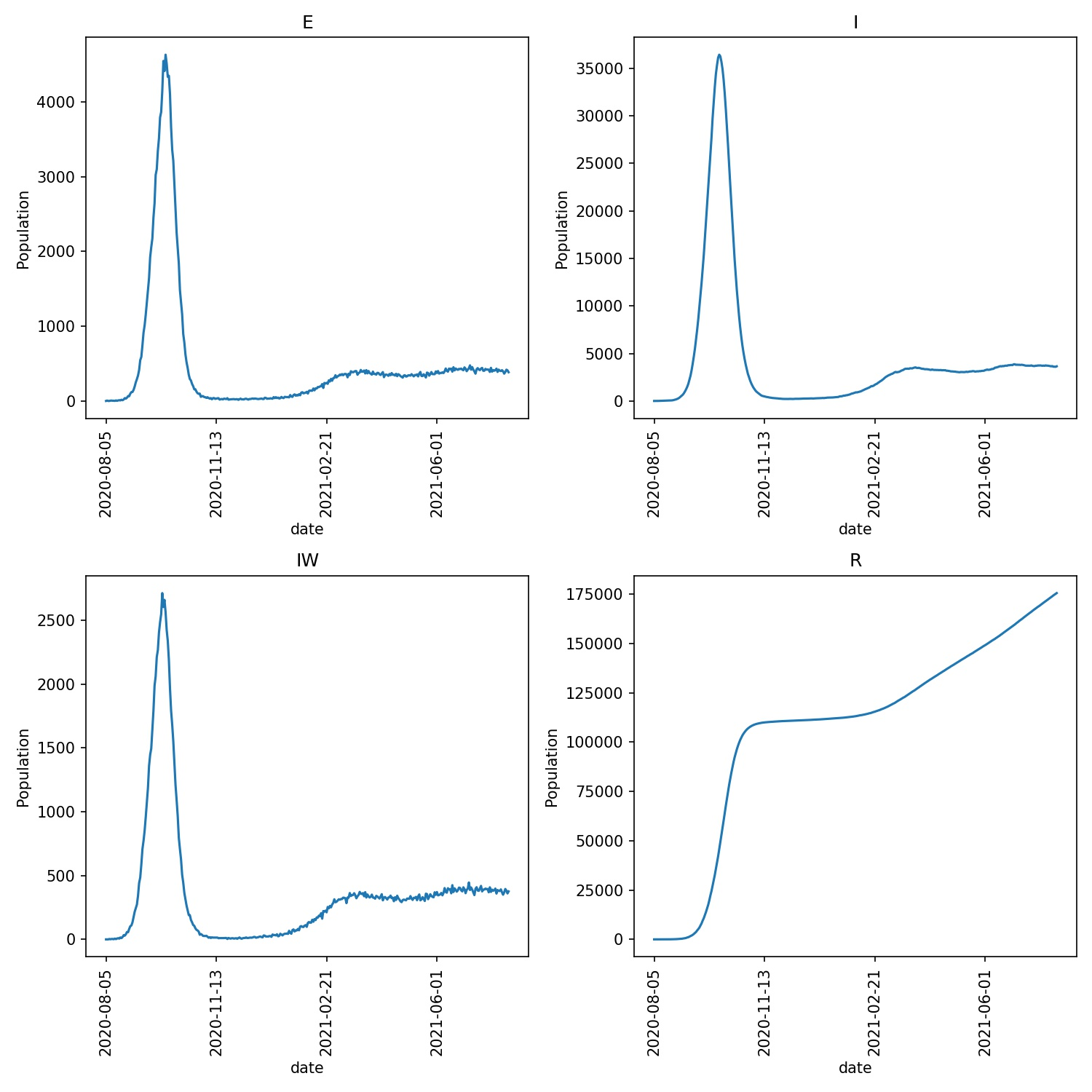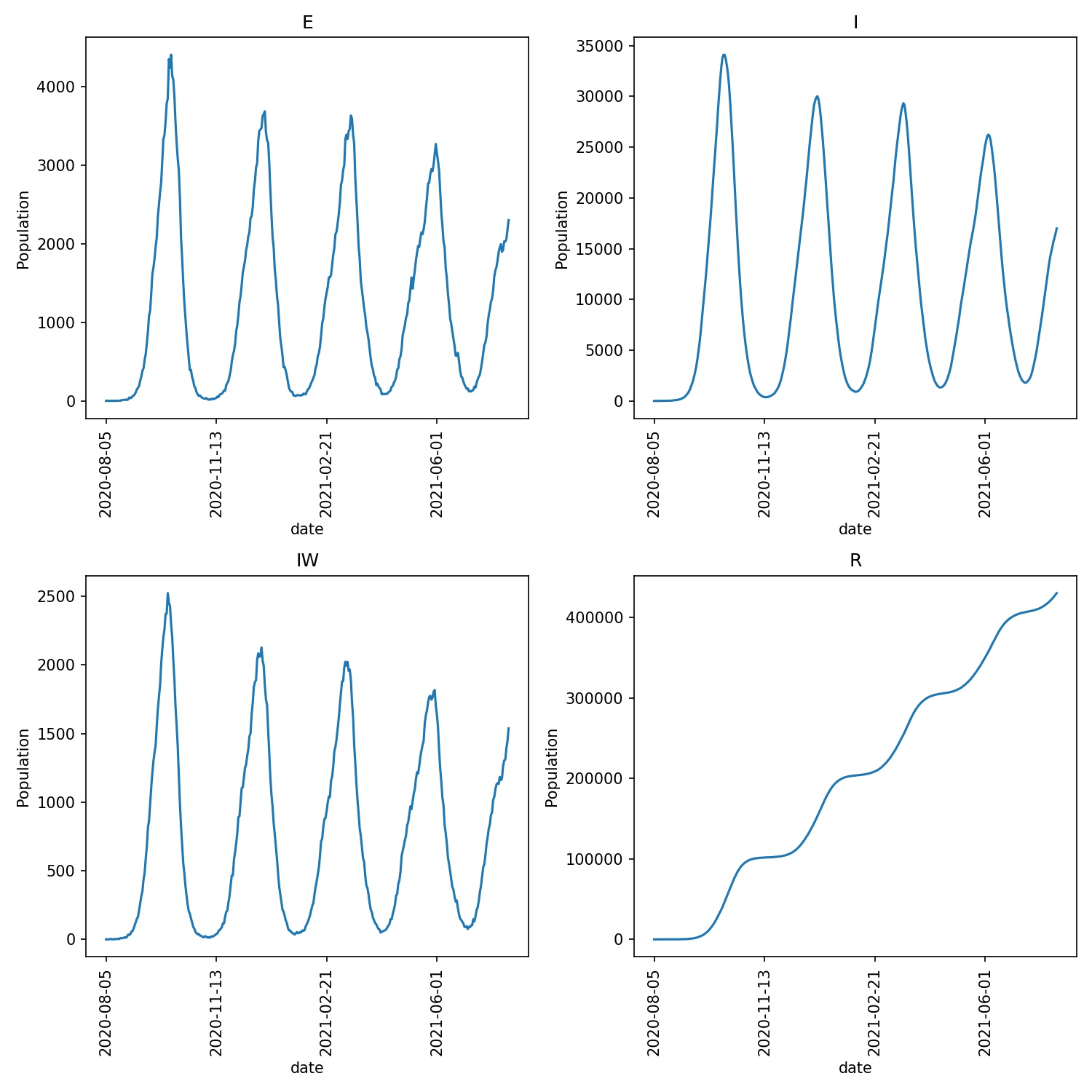# Local lockdowns

In the last chapter it was implied that the parameters for equation 1, and the action of the cutoff parameter, were global, and applied to all wards equally. If this was true, then it would make it difficult for metawards to model ward-specific behaviour, such as local lockdowns, or differences in local behaviour. In reality, metawards provides support for ward-specific values of the scaling and cutoff parameters.

## Limiting movement at a local level

Every ward can have its own value of the cutoff parameter. Movement between two wards is only permitted if the distance between wards is less than the minimum of the cutoff distance of each ward, and the value of Parameters.dyn_dist_cutoff, e.g. if this condition is true;

1. $$D_\text{ij} < \text{min}( C_i, C_j, C_\text{global} )$$

where;

• $$D_\text{ij}$$ is the distance between the centres of wards $$i$$ and $$j$$,

• $$C_i$$ is the local cutoff parameter for ward $$i$$,

• $$C_j$$ is the local cutoff parameter for ward $$j$$, and

• $$C_\text{global}$$ is the global cutoff distance set via Parameters.dyn_dist_cutoff

## Scaling FOI at a local level

Every ward can have its own value of the FOI scaling parameter. In reality, equation 1 is actually;

1. $$F(w) = S \times S_l(w) \times U(t) \times \sum_s [ C_s \beta_s N_s(w) ]$$

where;

• $$S_l(w)$$ is the local scaling factor for ward $$w$$. This acts together with $$S$$, $$U(t)$$ and $$C_s$$ to give you a lot of control over how infectious individuals at each disease stage in each ward contribute to the ward’s FOI.

## Reading and writing local parameters

You can read and write local ward parameters from within a custom iterator. The local ward parameters are stored in the network.nodes object. This provides arrays which are indexed by ward ID (i.e. counting up from 1 to nnodes + 1).

network.nodes.scale_uv contains the $$S_l(w)$$ values for each node, while network.nodes.cutoff contains the cutoff values for each node (in kilometers).

The scaling factors for each node default to 1.0 (meaning no local scaling), while the cutoff defaults to a large distance that is greater than the distance between any two points on Earth (meaning thus no local cutoff).

As these values are used as part of the FOI calculation, they must be read and set during the foi stage of the model day. For example, create a new iterator called lockdown.py and copy in the below;

from metawards.iterators import iterate_default

scale_uv = network.nodes.scale_uv
cutoff = network.nodes.cutoff

# set a lockdown in even-numbered wards
for i in range(2, network.nnodes, 2):
scale_uv[i] = 0.0
cutoff[i] = 0.0

def iterate_lockdown(stage, **kwargs):
# get the default functions for this stage
funcs = iterate_default(stage, **kwargs)

if stage == "foi":
# make sure that advance_lockdown is called
# first in the foi stage
else:
return funcs


This iterator defines the advance_lockdown advance function. This simply loops over all even-numbered wards and sets network.nodes.scale_uv and network.nodes.cutoff to zero. In effect, this places half of the country into extreme lockdown, where the disease is unable to spread.

The iterate_lockdown function takes the stage parameter. This tells metawards that this iterator wants to specify the advance functions to call at different stages. By default, this returns the default advance functions for that stage (as returned by iterate_default()). For the foi stage, this return advance_lockdown before the default functions, thereby ensuring that advance_lockdown changes the network.nodes.scale_uv and network.nodes.cutoff ward-local parameters before they are used to calculate the force of infection of each ward.

You can run this iterator using;

metawards -d lurgy3 -a ExtraSeedsLondon.dat --iterator lockdown


You should see that the infection spreads to only half of the country, as the lurgy can only infect the half of the population that are resident of visiting wards that are not in complete lockdown. For example, I see;

...

─────────────────────────────────────────────── Day 67 ───────────────────────────────────────────────
S: 45526353  E: 871045  I: 5537877  R: 4146802  IW: 4293  POPULATION: 56082077
Number of infections: 6408922

─────────────────────────────────────────────── Day 68 ───────────────────────────────────────────────
S: 44593470  E: 901872  I: 5911611  R: 4675124  IW: 4294  POPULATION: 56082077
Number of infections: 6813483

─────────────────────────────────────────────── Day 69 ───────────────────────────────────────────────
S: 43632470  E: 932883  I: 6269338  R: 5247386  IW: 4294  POPULATION: 56082077
Number of infections: 7202221

...

────────────────────────────────────────────── Day 184 ───────────────────────────────────────────────
S: 28691853  E: 0  I: 2  R: 27390222  IW: 0  POPULATION: 56082077
Number of infections: 2

────────────────────────────────────────────── Day 185 ───────────────────────────────────────────────
S: 28691853  E: 0  I: 2  R: 27390222  IW: 0  POPULATION: 56082077
Number of infections: 2

────────────────────────────────────────────── Day 186 ───────────────────────────────────────────────
S: 28691853  E: 0  I: 0  R: 27390224  IW: 0  POPULATION: 56082077
Number of infections: 0
Ending on day 186


## Custom ward-local parameters

You can also read and write your own custom ward-local parameters. You do this by calling network.nodes.get_custom. For example;

my_params = network.nodes.get_custom("my_params", default=0.0)


will return the custom ward-local parameters called my_params. If these don’t exist, then they are created, with each ward given a default starting value specified by default (here 0.0). The return value is the array indexed by ward ID. This can be read and written in an identical way to network.nodes.scale_uv and network.nodes.cutoff.

Note

Custom ward-level parameters are always stored as an array of floating point numbers.

You can use custom parameters to store or manipulate extra ward-level data. For example, edit your lockdown.py iterator to read;

from metawards.iterators import iterate_default
from metawards.utils import Console

# get the ward-specific scaling and cutoff parameters
scale_uv = network.nodes.scale_uv
cutoff = network.nodes.cutoff

# get the custom parameter 'in_lockdown' which we will
# initialise to 0 (meaning false)
in_lockdown = network.nodes.get_custom("in_lockdown", default=0)

# count of number of case-free days per ward
case_free_days = network.nodes.get_custom("case_free_days", default=0)

# get the total number of infections from the workspace
I_in_wards = workspace.I_in_wards

# loop over all wards
for i in range(1, network.nnodes + 1):
# is this ward in lockdown?
if in_lockdown[i]:
# has the number of infections dropped to zero? If so,
# then leave lockdown
if I_in_wards[i] == 0:
# we need 28 case-free days before releasing lockdown
if case_free_days[i] > 28:
Console.debug(f"Ward {i} leaving lockdown")
# stay on high vigilence, so keep actions that
# reduce beta to 20% of normal
scale_uv[i] = 0.2
cutoff[i] = 99999.99
in_lockdown[i] = 0
case_free_days[i] = 0
else:
case_free_days[i] += 1
Console.debug(f"Ward {i} case_free_days equals {case_free_days[i]}")
else:
case_free_days[i] = 0

# if not, then enter lockdown if the number of infections
# goes above 5
elif I_in_wards[i] > 5:
Console.debug(f"Ward {i} entering lockdown")
in_lockdown[i] = 1
case_free_days[i] = 0

# stop all travel and enact measures that
# will scale down beta to 1% of normal
cutoff[i] = 0.0
scale_uv[i] = 0.01

# get the number of wards in lockdown
num_lockdown = int(sum(in_lockdown))

if num_lockdown > 0:
Console.print(f"Number of wards in lockdown equals {num_lockdown}")

def iterate_lockdown(stage, **kwargs):
# get the default functions for this stage
funcs = iterate_default(stage=stage, **kwargs)

if stage == "foi":
else:
return funcs


In this case advance_lockdown will move individual wards in and out of local lockdowns depending on the number of infections in that ward (read from the workspace.I_in_wards array from the passed Workspace object).

Two ward-local custom parameters are used to record whether or not a ward is in a local lockdown;

• in_lockdown is 1 if the ward is in lockdown, and 0 if it is not

• case_free_days is the count of the number of consecutive days in a ward without an infection (really detectable infection, e.g. an individual in the I state).

The advance_lockdown function works by looping over all wards and seeing if the ward is in lockdown by checking the in_lockdown value for that ward. If it is, and if the number of infections is zero, then it checks if more than 28 case-free days have passed. If they have, then the local lockdown is relaxed, travel is allowed (cutoff is set to a large value) and the scaling factor is increased to 0.2 (implying that measures such as mask wearing, physical distancing etc. are still followed).

If 28 days have not passed, then the number of case-free days is incremented.

If the ward is not in local lockdown, then if the number of local detected infections goes above 5 then a local lockdown is initiated. Travel is halted (cutoff is set to 0) and stringent measures are taken such that the scaling factor is 0.01 (implying that beta is scaled down by 99%).

Finally, the number of wards in lockdown is calculated as the sum of the in_lockdown custom parameter, and is printed to the screen.

Note

Note that there are some additional Console.debug statements in the function that print out debug lines when wards move in and out of lockdown.

You can run this iterator using;

metawards -d lurgy3 -a ExtraSeedsLondon.dat --iterator lockdown


You should see that the number of wards in lockdown increases as the disease spreads. The spread is slowed down, but as wards come out of lockdown they are sometimes re-infected, and have to re-lockdown. You may see wave like behaviour as the disease is slowly brought under control. For example, for me, the plot of the outbreak, produced via;

metawards-plot -i output/results.csv.bz2


shows the following output/overview.jpg plot;This wave behaviour is more clear if we make the entering of exiting of local lockdown more extreme. For example, update your lockdown.py to read;

from metawards.iterators import iterate_default
from metawards.utils import Console

# get the ward-specific scaling and cutoff parameters
scale_uv = network.nodes.scale_uv
cutoff = network.nodes.cutoff

# get the custom parameter 'in_lockdown' which we will
# initialise to 0 (meaning false)
in_lockdown = network.nodes.get_custom("in_lockdown", default=0)

# count of number of case-free days per ward
case_free_days = network.nodes.get_custom("case_free_days", default=0)

# get the total number of infections from the workspace
I_in_wards = workspace.I_in_wards

# loop over all wards
for i in range(1, network.nnodes + 1):
# is this ward in lockdown?
if in_lockdown[i]:
# has the number of infections dropped to zero? If so,
# then leave lockdown
if I_in_wards[i] == 0:
# we need 28 case-free days before releasing lockdown
if case_free_days[i] > 28:
Console.debug(f"Ward {i} leaving lockdown")
# completely relax the lockdown
scale_uv[i] = 1.0
cutoff[i] = 99999.99
in_lockdown[i] = 0
case_free_days[i] = 0
else:
case_free_days[i] += 1
Console.debug(f"Ward {i} case_free_days equals {case_free_days[i]}")
else:
case_free_days[i] = 0

# if not, then enter lockdown if the number of infections
# goes above 5
elif I_in_wards[i] > 5:
Console.debug(f"Ward {i} entering lockdown")
in_lockdown[i] = 1
case_free_days[i] = 0

# stop all travel and enact measures that
# stop all local transmission (beta is 0)
cutoff[i] = 0.0
scale_uv[i] = 0.0

# get the number of wards in lockdown
num_lockdown = int(sum(in_lockdown))

if num_lockdown > 0:
Console.print(f"Number of wards in lockdown equals {num_lockdown}")

def iterate_lockdown(stage, **kwargs):
# get the default functions for this stage
funcs = iterate_default(stage=stage, **kwargs)

if stage == "foi":

The only change is that scale_uv[i] is set to 0.0 for wards that are in lockdown (i.e. there is no more spread), while scale_uv[i] is returned to 1.0 for wards that leave lockdown. This extreme switching when entering and leaving lockdown causes waves of infection that spread across wards, e.g. when I run this model I see;